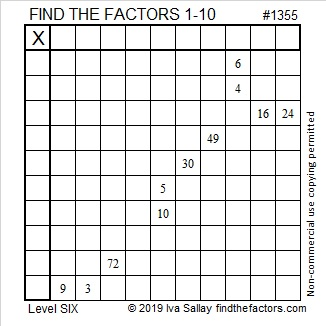# 1355 I Can Solve This Puzzle, Can You?

Level 6 puzzles are a little tricky because there is more than one possible common factor for every set of clues on the same row or column.

You can still solve it using logic. I can solve it. Can you?Print the puzzles or type the solution in this excel file: 10 Factors 1347-1356

If you need some logical hints, the video below will be helpful:

Now I’ll share some information about the puzzle number, 1355:

• 1355 is a composite number.
• Prime factorization: 1355 = 5 × 271
• 1355 has no exponents greater than 1 in its prime factorization, so √1355 cannot be simplified.
• The exponents in the prime factorization are 1, and 1. Adding one to each exponent and multiplying we get (1 + 1)(1 + 1) = 2 × 2 = 4. Therefore 1355 has exactly 4 factors.
• The factors of 1355 are outlined with their factor pair partners in the graphic below.1355 is the hypotenuse of a Pythagorean triple:
813-1084-1355 which is (3-4-5) times 271